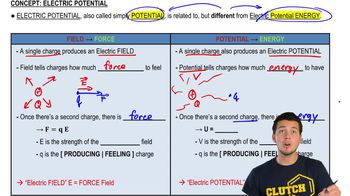Start typing, then use the up and down arrows to select an option from the list.
5:26 minutes
Problem 23c
Textbook Question

# Two point charges of equal magnitude Q are held a distance d apart. Consider only points on the line passing through both charges. (a) If the two charges have the same sign, find the location of all points (if there are any) at which (i) the potential (relative to infinity) is zero (is the electric field zero at these points?), and (ii) the electric field is zero (is the potential zero at these points?).Verified Solution
This video solution was recommended by our tutors as helpful for the problem above.
66views